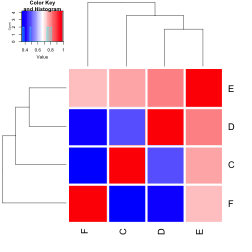##Install dependencies for Demonstration

``````library('mvtnorm')
library("igraph")
library("gplots")
library("graphsim")
``````

##set up simulated graphs

``````graph_test2_edges <- rbind(c("C", "E"), c("D", "E"), c("E", "F"))
graph_test2 <- graph.edgelist(graph_test2_edges, directed = T)
``````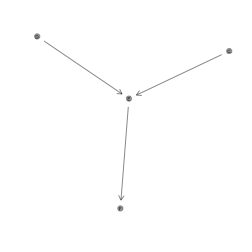#Generated simulated expression data from graph

``````adj_mat <- make_adjmatrix_graph(graph_test2)
heatmap.2(make_adjmatrix_graph(graph_test2), scale = "none", trace = "none", col = colorpanel(3, "grey75", "white", "blue"), colsep = 1:4, rowsep = 1:4)
``````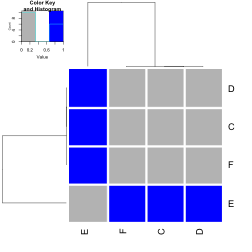``````heatmap.2(make_adjmatrix_graph(graph_test2, directed = T), scale = "none", trace = "none", col = colorpanel(3, "grey75", "white", "blue"), colsep = 1:4, rowsep = 1:4)
``````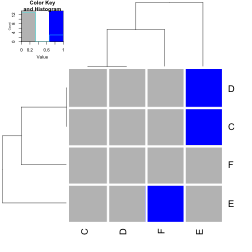``````comm_mat <- make_commonlink_graph(graph_test2)
heatmap.2(make_commonlink_graph(graph_test2), scale = "none", trace = "none", col = bluered(50), colsep = 1:4, rowsep = 1:4)
``````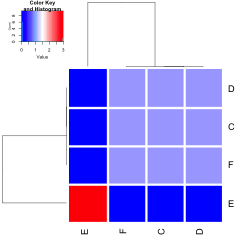##Distance matrix

``````shortest.paths(graph_test2)
``````
``````##   C E D F
## C 0 1 2 2
## E 1 0 1 1
## D 2 1 0 2
## F 2 1 2 0
``````
``````heatmap.2(shortest.paths(graph_test2), scale = "none", trace = "none", col = bluered(50), colsep = 1:4, rowsep = 1:4)
``````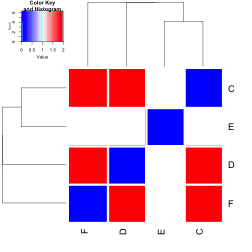``````(diameter(graph_test2)-shortest.paths(graph_test2))/diameter(graph_test2)
``````
``````##     C   E   D   F
## C 1.0 0.5 0.0 0.0
## E 0.5 1.0 0.5 0.5
## D 0.0 0.5 1.0 0.0
## F 0.0 0.5 0.0 1.0
``````
``````heatmap.2((diameter(graph_test2)-shortest.paths(graph_test2))/diameter(graph_test2), scale = "none", trace = "none", col = bluered(50), colsep = 1:4, rowsep = 1:4)
``````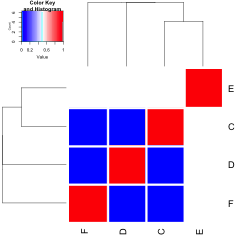``````make_distance_graph(graph_test2, absolute = F)
``````
``````##      C   E    D    F
## C 1.00 0.5 0.25 0.25
## E 0.50 1.0 0.50 0.50
## D 0.25 0.5 1.00 0.25
## F 0.25 0.5 0.25 1.00
``````
``````make_distance_graph(graph_test2, absolute = T)
``````
``````##     C   E   D   F
## C 1.0 0.5 0.0 0.0
## E 0.5 1.0 0.5 0.5
## D 0.0 0.5 1.0 0.0
## F 0.0 0.5 0.0 1.0
``````

##Sigma matrix

``````#sigma from adj mat
make_sigma_mat_graph(graph_test2, 0.8)
``````
``````##     C   E   D   F
## C 1.0 0.8 0.0 0.0
## E 0.8 1.0 0.8 0.8
## D 0.0 0.8 1.0 0.0
## F 0.0 0.8 0.0 1.0
``````
``````heatmap.2(make_sigma_mat_graph(graph_test2, 0.8), scale = "none", trace = "none", col = bluered(50), colsep = 1:4, rowsep = 1:4)
``````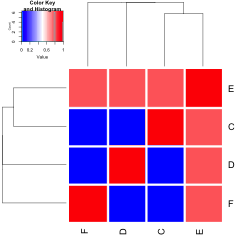``````#sigma from comm mat
make_sigma_mat_graph(graph_test2, 0.8, comm = T)
``````
``````##     C E   D   F
## C 1.0 0 0.8 0.8
## E 0.0 1 0.0 0.0
## D 0.8 0 1.0 0.8
## F 0.8 0 0.8 1.0
``````
``````heatmap.2(make_sigma_mat_graph(graph_test2, 0.8, comm = T), scale = "none", trace = "none", col = bluered(50), colsep = 1:4, rowsep = 1:4)
``````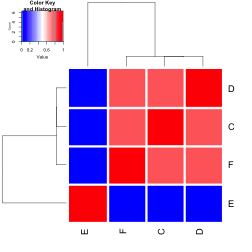``````# sigma from distance matrix
make_sigma_mat_dist_graph(graph_test2, 0.8, absolute = T)
``````
``````##     C   E   D   F
## C 1.0 0.8 0.0 0.0
## E 0.8 1.0 0.8 0.8
## D 0.0 0.8 1.0 0.0
## F 0.0 0.8 0.0 1.0
``````
``````make_sigma_mat_dist_graph(graph_test2, 0.8, absolute = F)
``````
``````##     C   E   D   F
## C 1.0 0.8 0.4 0.4
## E 0.8 1.0 0.8 0.8
## D 0.4 0.8 1.0 0.4
## F 0.4 0.8 0.4 1.0
``````
``````heatmap.2(make_sigma_mat_dist_graph(graph_test2, 0.8, absolute = T), scale = "none", trace = "none", col = bluered(50), colsep = 1:4, rowsep = 1:4)
````````````heatmap.2(make_sigma_mat_dist_graph(graph_test2, 0.8, absolute = F), scale = "none", trace = "none", col = bluered(50), colsep = 1:4, rowsep = 1:4)
``````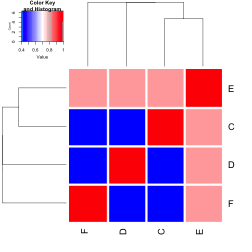#Simulated expression and observed correlation

``````#simulate expression data
expr <- generate_expression(100, graph_test2, cor = 0.8, mean = 0, comm =F) # unable to generate from adj mat ## fixed with positive definite correction
``````
``````## Warning in generate_expression(100, graph_test2, cor = 0.8, mean = 0, comm = F):
## sigma matrix was not positive definite, nearest approximation used.
``````
``````heatmap.2(expr, scale = "none", trace = "none", col = bluered(50), colsep = 1:4, rowsep = 1:4)
``````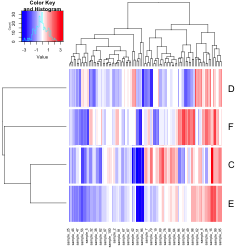``````heatmap.2(cor(t(expr)), scale = "none", trace = "none", col = bluered(50), colsep = 1:4, rowsep = 1:4)
``````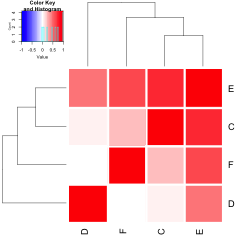``````#comm mat
expr <- generate_expression(100, graph_test2, cor = 0.8, mean = 0, comm =T) #expression from comm mat
heatmap.2(expr, scale = "none", trace = "none", col = bluered(50), colsep = 1:4, rowsep = 1:4)
``````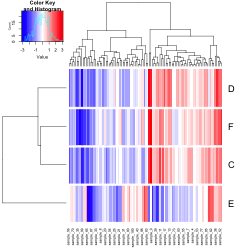``````heatmap.2(cor(t(expr)), scale = "none", trace = "none", col = bluered(50), colsep = 1:4, rowsep = 1:4)
``````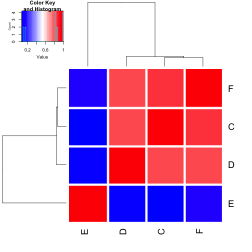``````#absolute dist
expr<- generate_expression(100, graph_test2, cor = 0.8, mean = 0, comm = F, dist = T, absolute = T) # unable to generate from adj mat ## fixed PD
``````
``````## Warning in generate_expression(100, graph_test2, cor = 0.8, mean = 0, comm =
## F, : sigma matrix was not positive definite, nearest approximation used.
``````
``````heatmap.2(expr, scale = "none", trace = "none", col = bluered(50), colsep = 1:4, rowsep = 1:4)
``````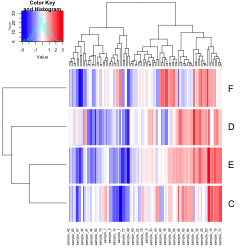``````heatmap.2(cor(t(expr)), scale = "none", trace = "none", col = bluered(50), colsep = 1:4, rowsep = 1:4)
``````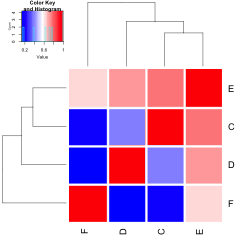``````# relative dist
expr<- generate_expression(100, graph_test2, cor = 0.8, mean = 0, comm = F, dist = T, absolute = F)
``````
``````## Warning in generate_expression(100, graph_test2, cor = 0.8, mean = 0, comm =
## F, : sigma matrix was not positive definite, nearest approximation used.
``````
``````heatmap.2(expr, scale = "none", trace = "none", col = bluered(50), colsep = 1:4, rowsep = 1:4)
``````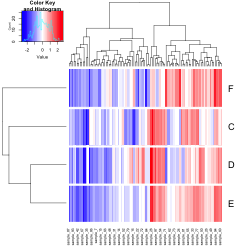``````heatmap.2(cor(t(expr)), scale = "none", trace = "none", col = bluered(50), colsep = 1:4, rowsep = 1:4)
``````